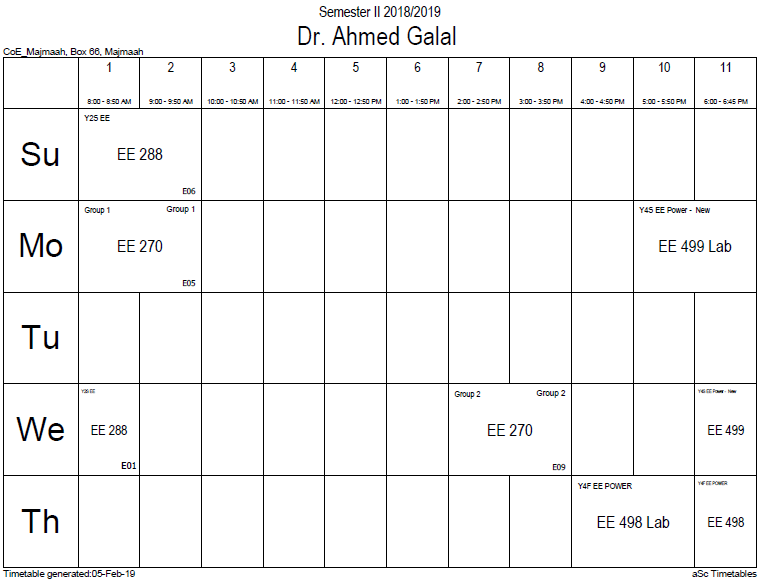## Force between 2cyl

For two cylindrical magnets with radius$R$ and height$t$, with their magnetic dipole aligned, the force can be well approximated (even at distances of the order of$t$) by,$F(x) = frac{pimu_0}{4} M^2 R^4 left[frac{1}{x^2} + frac{1}{(x+2t)^2} - frac{2}{(x + t)^2} ight]$

where$M$ is the magnetization of the magnets and$x$ is the distance between them. In disagreement to the statement in the previous section, a measurement of the magnetic flux density very close to the magnet$B_0$ is related to$M$ by the formula$B_0 = mu_0 M$

The effective magnetic dipole can be written as$m = M V$

Where$V$ is the volume of the magnet. For a cylinder, this is$V = pi R^2 t$.

When$t << x$, the point dipole approximation is obtained,$F(x) = frac{3pimu_0}{2} M^2 R^4 t^2frac{1}{x^4} = frac{3mu_0}{2pi} M^2 V^2frac{1}{x^4} = frac{3mu_0}{2pi} m_1 m_2frac{1}{x^4}$

which matches the expression of the force between two magnetic dipoles.

### Office HoursNo office hours### My Timetable### Contactsemail: [email protected]

Phone: 2570

### Welcome

Welcome To Faculty of Engineering### IEEEhttp://www.ieee.org/

/

### Bookmarkshttp://www.utk.edu/research/

http://science.doe.gov/grants/index.asp

http://www1.eere.energy.gov/vehiclesandfuels/

http://www.eere.energy.gov/

### Upcoming Conferences### Engineering quotes### Travel Web Siteshttp://www.hotels.com/

http://www.orbitz.com/

http://www.hotwire.com/us/index.jsp

http://www.kayak.com/

### Blackboardستقام اختبارات الميدتيرم يوم الثلاثاء 26-6-1440

حسب الجدول المعلن بلوحات الاعلان

### Summer trainingThe registration for summer training will start from 5th week of second semesterClass registration week 1

### برنامج التجسير### إحصائية الموقع

عدد الصفحات: 2879

البحوث والمحاضرات: 1280

الزيارات: 99483